Mobile QR Code1. (Department of Electrical and Electronic Engineering, Hannam University)

Digital background calibration, pipelined SAR ADC, gradient decent algorithm, ultrasound, FPGA implementation

## I. INTRODUCTION

Fig. 1. (a) Ultrasound transceiver for Doppler processing, (b) a conceptual spectrum of ultrasonic echo signal.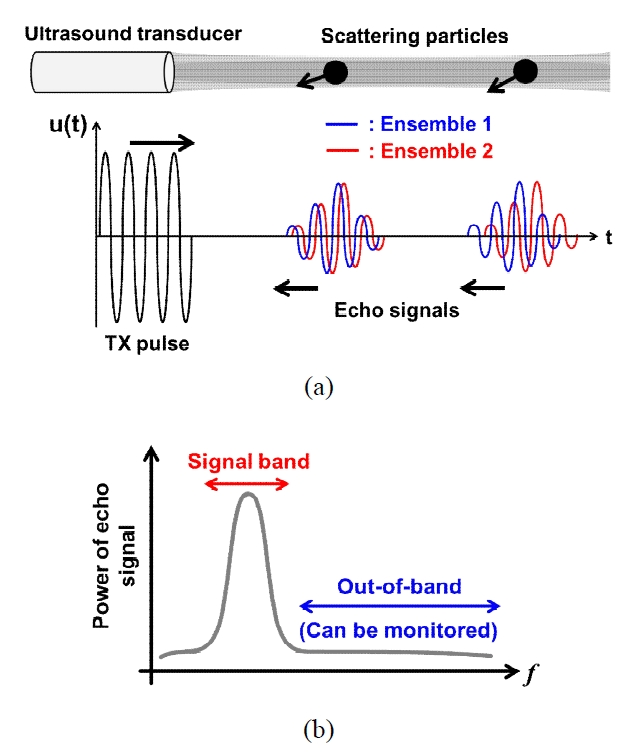Typically, the application of ultrasonic Doppler signal processing utilizes an ultrasound transducer with a specific center frequency by a nature of transducer element. So, an acquired ultrasonic echo signal has a narrow frequency band with a specific center frequency. (Fig.1). In addition, there is a sampling frequency requirement, i.e., 8 to 16 times of center frequency of transducer, to relieve hardware complexity in the following signal-processing unit such as a beamformer [1,2]. This is because a delay resolution of a beamformer is primarily determined by an oversampling ratio (OSR) of an analog-to-digital converter (ADC) [1,2].

This work proposes an ultrasonic echo-signal based digital background calibration for pipelined successive approximation register (SAR) ADC. It utilizes the abovementioned features of target application, ultrasonic Doppler processing. The proposed ADC periodically monitors a power of out-of-band signals (Fig.1(b)). The power of out-of-band high-frequency signals correspond to harmonics, which are generated by nonlinearity of ADC. The proposed calibration corrects the nonlinearity by minimizing the power of harmonics with an optimization algorithm.

The target resolution and sampling rate of ADC in this work are 10 bits and 11 MS/s, respectively. A SAR ADC can be preferred structure for those specifications in terms of energy efficiency. However, the total input capacitance of SAR ADC becomes large if the utilized CMOS process does not belong to a few tens of \textit{nm} CMOS process. This results in a large capacitive loading for an ADC driver circuit. When we consider the available minimum metal-insulator-metal (MIM) capacitance in the utilized CMOS process, we choose a pipelined-SAR ADC structure to compromise the energy efficiency and the input capacitive loading [3,4].

The resolution of multi-step (pipeline-type) ADC is primarily affected by the inter-stage gain error resulting from capacitance mismatch, finite op-amp gain, charge sharing in top plate of capacitive digital-to-analog converter (DAC), and a utilization of open-loop amplifier.

There are various approaches to mitigate inter-stage gain error of multi-step ADCs [5-10]. A pseudo-random noise (PN) sequence has been utilized in digital calibration for error randomization, and error code is detected through a correlation-based method [5,6]. This method is usually applied in a digital background calibration. However, it can reduce a signal dynamic range due to an injection of PN sequence in a signal path. Also, PN-sequence-based calibration requires relatively long convergence time to detect a PN-modulated error.

Histogram-based digital calibration [7,8] is an another approach to compensate for gain error. It accumulates a code distribution. Then, specific code statistics, i.e., code distance or width, are extracted to estimate error code. Since it depends on an accumulated code histogram, rigorous requirements are imposed on an input signal of ADC for calibration accuracy.

Several foreground calibrations of [9,10] are feasible approaches to alleviate the gain error of pipelined SAR ADC. These calibration schemes commonly use an additional tunable capacitor bank. The accuracy of calibration can be affected by the precision of additional capacitor bank. Also, by a nature of a foreground calibration, it cannot track long-term process variations unless an intermittent calibration cycle is considered.

In this work, an ultrasonic echo-signal based digital background calibration for pipelined SAR ADC is proposed. This work utilizes a normal ultrasonic echo signal, and adaptively compensates for a periodic inter-stage gain error by using a gradient-decent optimization algorithm. As a result, there is no reduction of input-signal dynamic range, which is present in PN-based dithering. Also, there is no stringent constraint for a precision of input signal, compared with histogram-based method. The abovementioned calibrations such as PN-based method, histogram-based method and foreground calibrations require analog-circuit modifications on a signal path to measure error value directly. Whereas, this work does not require other analog-circuit modifications, whose imperfection normally affects the performance of calibration.

This paper is organized as follows. Section II presents the structure and issues of the pipelined SAR ADC. Section III presents the concept and implementation results for digital calibration. Measurement results and conclusion are followed in Section IV and V, respectively.

## II. STRUCTURE OF PIPELINED SAR ADC

Fig.2 shows a structure of two-stage pipelined SAR ADC. Each stage utilizes the merged capacitor switching (MCS) based SAR ADC . A residue voltage of the first stage is amplified by a residue amplifier. To enhance energy efficiency of ADC, a class-AB telescopic op amp is utilized as the residue amplifier . Building blocks of Fig.2 are implemented as a fully differential circuitry, and single-ended fashion is shown in Fig.2 for simplicity.

The first stage of Fig.2 is a 3.5-bit/stage multiplying digital-to-analog converter (MDAC) and the second stage is a 7-bit SAR quantizer. The raw output code $\textit{D}$$_{RAW} is generated by the conventional digital correction scheme . The nonlinearity of raw code \textit{D}$$_{RAW}$ is corrected by the proposed digital background calibration, which is described in Section III. The first-stage MDAC is implemented using MIM capacitors with unit capacitance of 20 fF. The second-stage SAR quantizer is implemented using full-custom designed metal-oxide-metal (MOM) capacitors  for unit capacitance of 1 fF.

Table 1. Design considerations of ADC in this work

 Consideration Countermeasure Power efficiency Class-AB topology in op amp Top-plate sampling in DAC Capacitor mismatch Common-centroid layout Switch nonlinearity Bootstrapping scheme Fully differential topology Finite op-amp gain Telescopic topology in op amp Inter-stage gain error Proposed digital calibration

There are primary design considerations in a pipelined SAR ADC (Table 1). This work deals with each of design considerations with appropriate design techniques. Power efficiency is one of significant considerations, and this work adopts a class-AB op amp and top-plate sampling scheme in DAC. When we consider the target linearity, i.e., around 9 bits, a typical common-centroid layout of capacitive DAC can attain the target linearity. The class-AB telescopic op amp with DC gain more than 70 dB is used. Also, a bootstrapping scheme of sampling switches and fully differential topology in a signal path could alleviate a switch nonlinearity.

As is well known, a bottom-plate sampling SAR quantizer has an advantage that the parasitic capacitor of the output node of DAC does not affect the charge redistribution of DAC. However, the bottom-plate sampling SAR quantizer has more number of switches, which are connected to the bottom node of each capacitor of DAC. Correspondingly, a parasitic capacitance along with junction capacitances at the bottom plate of each capacitor can be increased. This un-avoidable parasitic capacitance can slow down settling speed of DAC, so a size of switch in bottom node of DAC capacitor might be increased. However, this increment of switch size worsens power consumption and chip area if the utilized CMOS process does not belong to advanced scaled CMOS process.

This work adopts the MCS with top-plate sampling scheme as a sampling and switching scheme. Also, this can reduce a load capacitance of the residue amplifier. However, the charge redistribution of top-plate sampling scheme is affected by a parasitic capacitor at the output node of DAC . This results in a gain error in the transfer function of DAC and residue-voltage error in the output of residue amplifier. The parasitic capacitance at the output node of DAC is mostly generated by routing lines in layout and an input capacitance of a comparator.

Note that capacitance matching characteristic of MIM capacitor is assumed to be enough to accomplish the linearity up to 9 effective number of bits (ENOB). Also, the open-loop gain of residue amplifier is 79 dB for typical corner condition in post-layout simulation. Hence, capacitance matching of DAC and op-amp gain can be considered to be negligible error sources in this work.

Then, the remained primary error source can be the reference scaling due to parasitic capacitance at the output node of DAC. The equivalent reference level for each stage can be summarized as follows :

##### (1)
$$V_{R E F . E Q}=\frac{C_{t o t a l}}{C_{P}+C_{t o t a l}} V_{R E F}$$

where $\textit{C}$$_{total} and \textit{C}$$_{P}$ correspond to a summation of DAC capacitors including dummy capacitor and a parasitic capacitance of DAC output, respectively.

As shown in (1), the equivalent reference level $\textit{V}$$_{REF.EQ} is scaled due to the parasitic capacitance of DAC output. The equivalent reference level \textit{V}$$_{REF.EQ}$ of each of stages cannot be accurately matched because it is difficult to precisely adjust a parasitic capacitance of DAC output. As a result, a discrepancy of reference levels between two adjacent stages occurs.

Fig. 3. (a) Reference-voltage discrepancy, (b) corresponding ADC transfer function.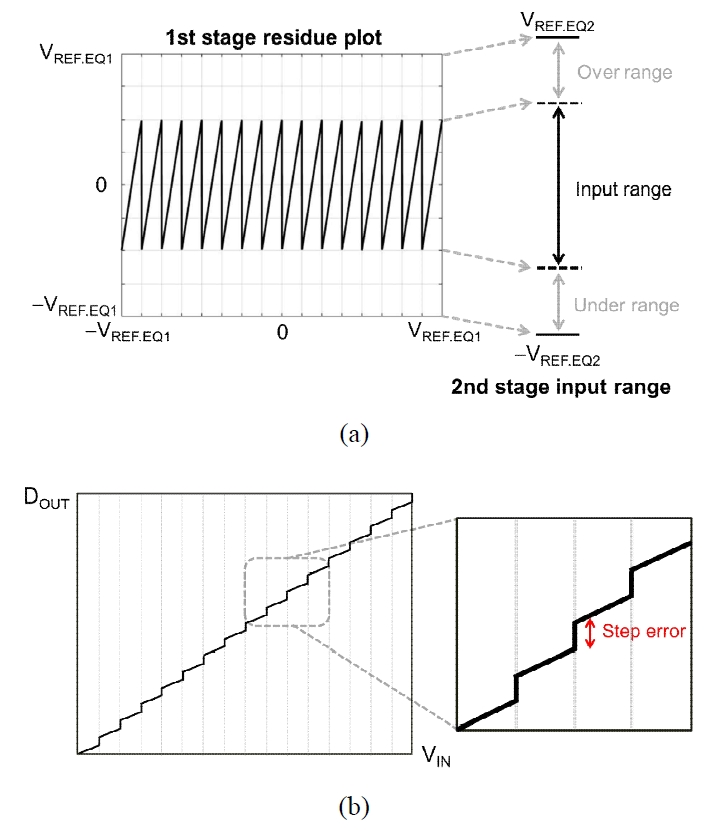The reference-voltage discrepancy is conceptually illustrated in Fig.3(a). This reference-voltage discrepancy causes a step error between two adjacent code segments as shown in Fig.3(b). The step error of ADC-transfer function results in nonlinearity components in the output of ADC. This nonlinearity of ADC generates harmonics of the input signal of ADC. If the harmonics power is suppressed by adjusting the step error in the digital domain, then the linearity of ADC can be enhanced. Therefore, this work focuses on the digital calibration scheme to track the step error in the digital domain.

## III. DIGITAL BACKGROUND CALIBRATION

### 1. Concept of Calibration

Fig. 4. (a) Block diagram of digital calibration, (b) gradientdecent optimization to track the step error.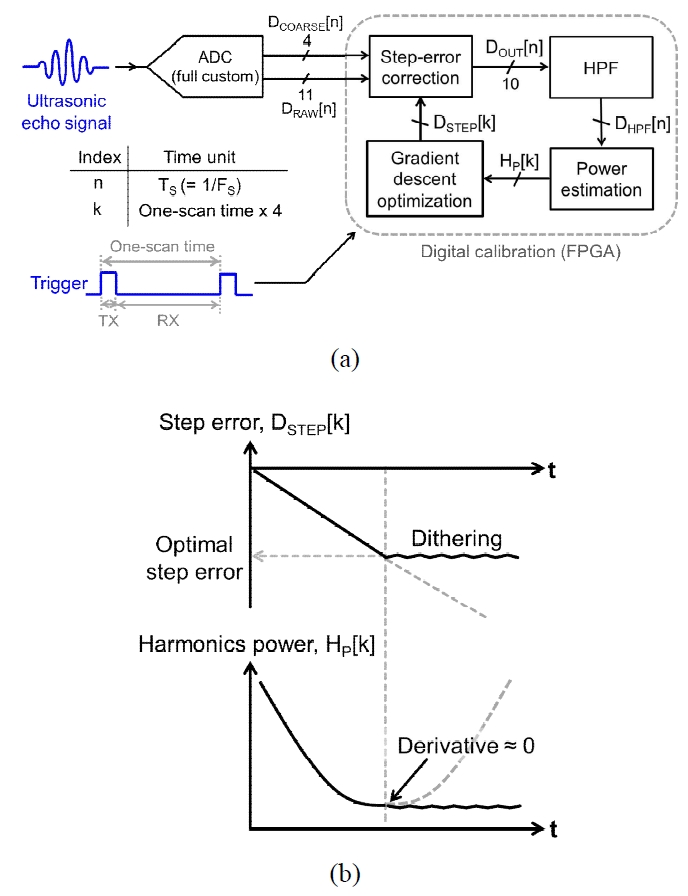Fig. 5. (a) Conceptual operation of step-error correction, (b) block diagram of step-error correction.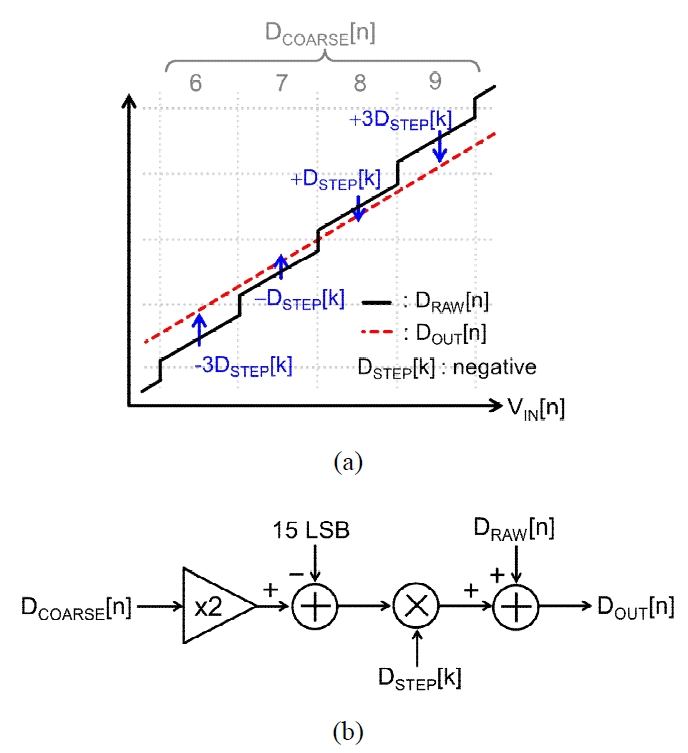The concept of proposed calibration is to minimize the harmonics power by adaptively adjusting the step error between coarse-code segments. This means it requires an algorithm to find the minimum value of a function. One of widely utilized algorithms to find the local minimum is a gradient-decent algorithm [15,16]. We can assume a function of harmonics power has a single minimum value, since the dominant error source of ADC is considered to be the periodic step error in this work. So, this work utilizes the gradient-decent optimization algorithm to track the minimum value of harmonics power.

Fig.4(a) presents a block diagram for digital background calibration. The digital calibration part consists of a step-error correction block, a high-pass filter (HPF), a power estimation block, and a gradient-decent optimization block.

When the raw code $\textit{D}$$_{RAW}[\textit{n}] is fed into the step-error correction block, the code correction is performed with the estimated step error \textit{D}$$_{STEP}$[$\textit{k}$]. The index $\textit{n}$ follows the time unit of $\textit{T}$$_{S}, which corresponds to the period of sampling clock. The index \textit{k} represents a time unit, whose period corresponds to four times of one-scan time (Fig.4(a)). The output code \textit{D}$$_{OUT}$[$\textit{n}$] of step-error correction block is delivered to HPF to extract harmonics signal. Note that the primary application of this work is an ultrasound Doppler processing. So, the echo signal has a relatively high Q factor. Also, the target carrier frequency of ultrasound is relatively low compared with the sampling frequency of ADC. Correspondingly, the utilization of HPF is relevant to evaluate harmonics power of ADC. The harmonics power is estimated by the power estimation block. The estimated power value $\textit{H}$$_{P}[\textit{k}] is utilized by the gradient-descent optimization block. This block tracks the optimal step error \textit{D}$$_{STEP}$[$\textit{n}$] which makes the derivative of harmonics power $\textit{H}$$_{P}[\textit{n}] zero (Fig.4(b)). ### 2. Implementation Fig. 6. (a) Block diagram of Butterworth 8-th order HPF, (b) magnitude response of HPF with and without quantization of filter coefficient.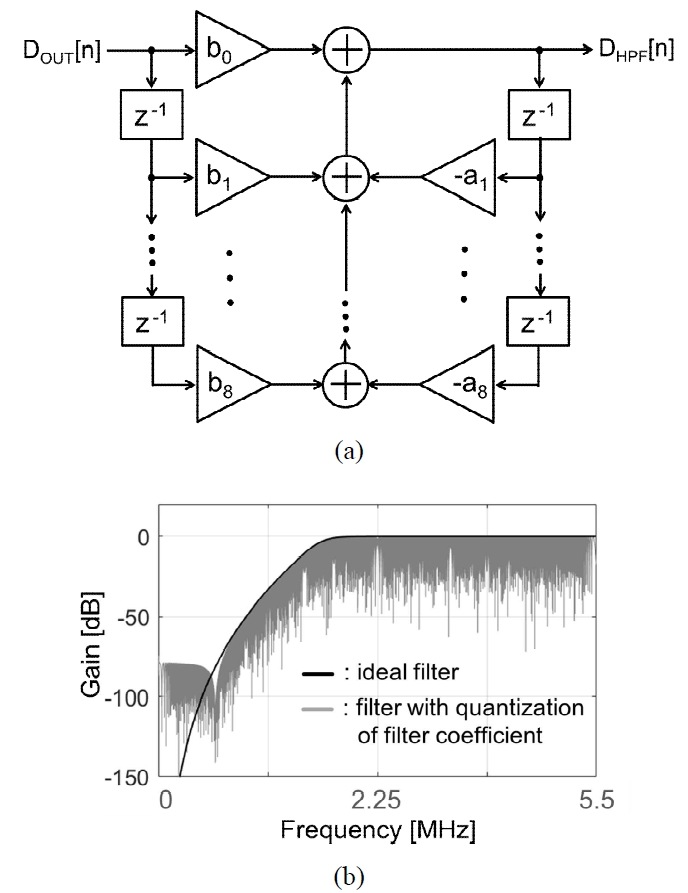Fig. 7. (a) Block diagram of power estimation, (b) block diagram of gradient-decent optimization algorithm.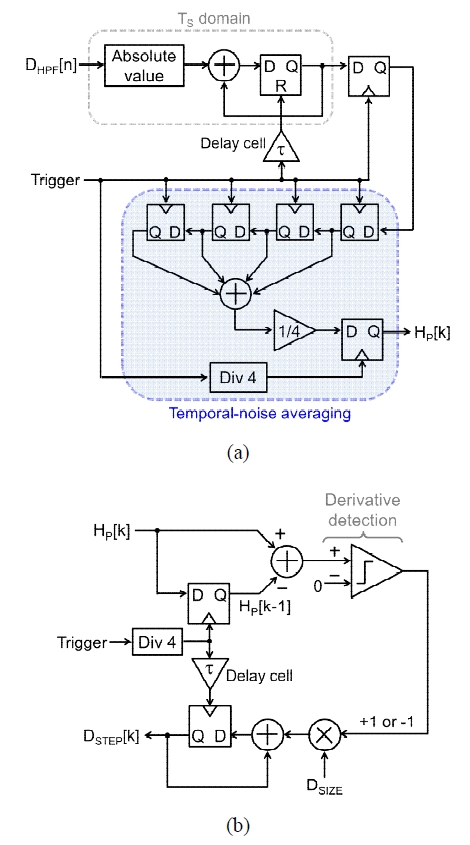The digital background calibration (Fig.4(a)) of this work is implemented with hardware description language (HDL), Verilog. The verification of HDL implement-tation has been performed by using a FPGA, Virtex 5 (Xilinx). A conceptual step-error correction is illustrated in Fig.5(a). According to the characteristic of residue plot of the 1st-stage MDAC, the step error between adjacent code segments has a regular code gap. This work compensates for the regular code gap by moving each of code segments with respect to the center code of full-scale output of ADC. The code \textit{D}$$_{COARSE}$[$\textit{n}$] represents the code from the 1st- stage MDAC, and it corresponds to each of code segments (Fig.5(a)). So, the movement of code segment is performed according to the code of $\textit{D}$$_{COARSE}[\textit{n}]. This code correction is performed by using the block diagram of Fig.5(b). It consists of a floating-pint multiplier and two adders. Note that all digital computations are executed with twos complement format. The estimated step error \textit{D}$$_{STEP}$[$\textit{k}$] is weighted by 2·$\textit{D}$$_{COARSE}[\textit{n}] – 15 LSB. The code \textit{D}$$_{STEP}$[$\textit{k}$] is manipulated in 12 digits of floating point.

The output code $\textit{D}$$_{OUT}[\textit{n}] of step-error correction block is delivered into HPF to filter out harmonics component. The harmonics power is monitored to evaluate the nonlinearity of ADC. The utilized HPF is Butterworth 8-th order filter (Fig.6(a)). Note that the ultrasonic signal of this work has a center frequency of 550 kHz with a Q-factor around 5. Correspondingly, the cut-off frequency of HPF is set to 2.06 MHz. When we consider the hardware implementation of filter, the filter coefficient should be truncated into a finite number of floating points. This work utilizes filter coefficients with 12 bits of floating points. Then, the filter performance can be degraded. In order to evaluate the filter-performance deviation due to quantization of filter coefficient, we applied a chirp signal. A comparison of magnitude respond of HPF is shown in Fig.6(b). Since the number of bits of overall ADC output is 10 bits, the utilization of 12 bits of floating points in filter coefficient is sufficient to attenuate the low frequency signal more than 60 dB. The harmonics, i.e., output code of HPF, is fed into the power estimation block. A power of harmonics signal can be computed through RMS computation. However, RMS computation is relatively complicated in terms of HDL implementation. Hence, this work utilizes an accumulation of absolute amplitude of harmonics signal (Fig.7(a)). This simple implementation can accomplish an equivalent computation of signal power. For robust estimation of harmonics power against temporal noise of ADC code, an averaging operation is performed for four consecutive power values (Fig.7(a)). To support this temporal-noise averaging, the step error \textit{D}$$_{STEP}$[$\textit{k}$] and harmonics power $\textit{H}$$_{P}[\textit{k}] are updated according to a signal, which is a divided signal for the periodic signal Trigger. A goal of this work is to suppress harmonics power \textit{H}$$_{P}$[$\textit{k}$] by tracking the step-error code $\textit{D}$$_{STEP}[\textit{k}] with the gradient-decent optimization algorithm . This algorithm periodically checks a derivative of harmonics power, and it becomes a steady state if the derivative of harmonics power approaches to zero. Fig. 9. (a) Ultrasonic echo signal applied into fabricated chip, (b) comparison of measured spectrums.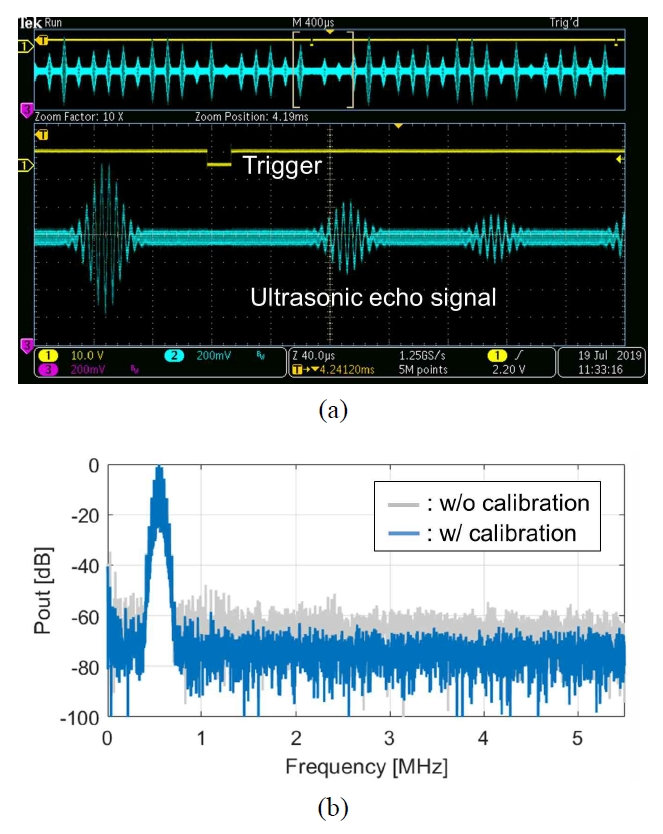This algorithm is implemented by using a block diagram of Fig.7(b). The polarity of derivative of harmonics power is computed by using a delay cell, a subtraction unit, and a comparator. There is an assumption that the raw code \textit{D}$$_{RAW}$[$\textit{n}$] of ADC has a transfer function of missing-code profile. Then, the step-error is decreased if the derivative of harmonics power is negative. Similarly, the step-error is increased if the derivative of harmonics power is positive. The step-error code $\textit{D}$$_{STEP}[\textit{k}] is decremented or incremented depending on the output of comparator. The step-error code \textit{D}$$_{STEP}$[$\textit{k}$] is updated with the following equation.

##### (2)
\begin{aligned} D_{S T E P}[k+1]=& D_{S T E P}[k] \\ &+\operatorname{sgn}\left(H_{P}[k]-H_{P}[k-1]\right) \cdot D_{S I Z E} \end{aligned}

where $\textit{D}$$_{SIZE} represent a step size for the utilized algorithm, and its value in the HDL implementation is 0.03125 LSB. Table 2. HDL synthesis result for implementation in FPGA.  Macro statistics Floating-point multiplier 18 x 18 1 24 x 24 9 33 x 24 8 Adder 13 bit 1 18 bit 2 24 bit 1 56 bit 20 Counter 10 bit 3 Register Flip-flop 1446 Slice logic utilization Slice LUT 2457 Register 1446 ## IV. MEASUREMENT RESULTS Fig. 11. Measured FFT results for (a) low-frequency signal, (b) Nyquist signal.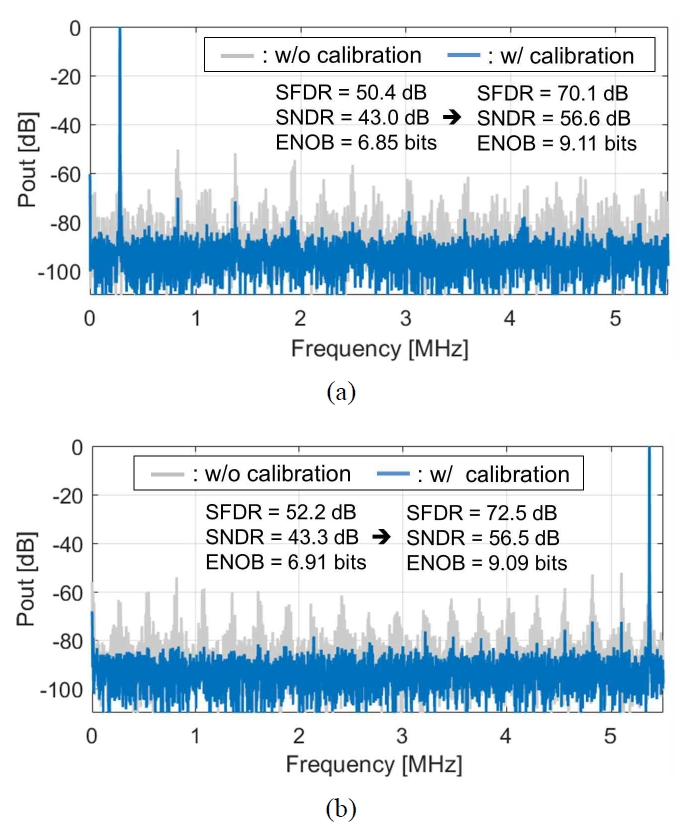The ADC core was implemented in a 0.18-μm standard CMOS process. The chip micrograph is shown in Fig.8. It includes the analog part of ADC and the digital part for raw code generation. The active area of fabricated circuit is 550 μm x 870 μm. The supply voltage of ADC is 1.8 V with power consumption of 530~μW. The power consumption of digital calibration is estimated to be 130 μW according to the synthesis result with Design Compiler (Synopsys). The sampling rate of ADC is 11 MS/s. In this work, the circuit of  was revised in terms of power consumption. In the revised circuit, the power consumption of residue amplifier was lowered, compared with that of . Also, the parasitic capacitance of the bottom plate of DAC capacitor was decreased through modified layout, and the DAC driver circuit was optimized for its DAC capacitor along with the bottom-plate parasitic capacitance. Through this circuit revision, the power consumption of ADC was lowered. The measurement boards consist of the FPGA board and the implemented PCB. The ultrasonic echo signal was emulated from the Field-II program . The emulated echo signal for a single scan line was applied to the fabricated ADC through a discrete DAC (DAC902E, Texas Instruments). The output of DAC was connected to the fabricated ADC. The ADC output codes, i.e., \textit{D}$$_{COARSE}$ and $\textit{D}$$_{RAW}, are fed into FPGA and manipulated with the proposed digital background calibration. The waveform of emulated ultrasonic echo signal is presented in Fig.9(a). The measured spectrums depending on proposed calibration are compared in Fig.9(b). Note that the proposed step-error correction compensates for the segmental offset, so it can affect the overall amplitude of signal after calibration. Thus, the spectrum of Fig.9(b) with calibration has been acquired after performing normalization of signal amplitude. A number of data sample for a single scan line is 2^{13}. In the FFT result, the harmonics of raw data of ADC are suppressed. Table 3. Performance comparison of ADCs with digital calibration  This work TCAS1'17  JSSC'09  JSTS'19  JSSC'12  ADC type Pipelined SAR Pipelined SAR Pipeline SAR Pipeline Calibration Background (off-chip, FPGA) Foreground (on chip) Foreground (off chip) Background (on chip) Background (off chip) Input for calibration Sensor signal (ultrasonic echo) Internally generated Reference DAC (DC signal) Sinusoidal Sinusoidal Resolution [bits] 10 12 10 11 12 Process [nm] 180 65 90 65 90 Supply [V] 1.8 1.2 1.2 0.7 1.0 Fs [MS/s] 11 160 500 5 30 Power [mW] 0.53 (0.66**) 6 55 0.073 2.95** SNDR[dB] @ Nyquist rate 56.5 60.9 52.8 53.5 64.5 FoM [fJ/conv-step] 110** 36.7 308* 37.5 72** * Power of digital calibration is excluded ** Estimated power of digital calibration is included To illustrate the operation of calibration, digital signals of step error \textit{D}$$_{STEP}$[$\textit{k}$] and harmonics power $\textit{H}$$_{P}$[$\textit{k}$] in FPGA are measured as shown in Fig.10. The harmonics power has its minimum value after tracking the optimum ep-error code (Fig.10).

This work demonstrates the implementation result of digital calibration by using HDL, Verilog, along with FPGA. The synthesis summary for implementation of designed HDL is shown in Table 2. Most of floating-point multipliers are utilized in the Butterworth 8-th order filter.

To demonstrate the linearity improvement of ADC, single-tone evaluations were performed. In this measurement, the step error was fixed into the tracked value from the ultrasonic-echo test. When a sinusoidal signal with a frequency of 275 kHz is applied, SNDR and SFDR are enhanced by 13.6 dB and 19.7 dB, respectively, after calibration. For a sinusoidal signal with Nyquist frequency, the achieved SNDR and SFDR are 56.5 dB and 72.5 dB, respectively. Through calibration, SNDR and SFDR are enhanced by 13.2 dB and 20.3 dB, respectively. As described before, the proposed calibration corrects a periodic inter-stage gain error, and correspondingly it suppresses spurs caused by the period inter-stage gain error. However, there are residual spurs in FFT spectrum after calibration. It is estimated that the remained nonlinearities might be caused by error sources which are not perfectly regulated with countermeasures of Table 1. The measured SNDR with respect to the input frequency are presented in Fig.12. The dynamic performance is maintained up to Nyquist input frequency (Fig.12).

The performance of this work is compared with other ADCs with digital calibration in Table 3. Most of ADCs in Table 3 require a relatively precise signal such as a sinusoidal or DC signal for calibration. However, this work uses an arbitrary sensor signal, i.e., ultrasonic echo, within pre-defined frequency range. However, the Walden FoM of this work is relatively higher than that of other works. This is estimated that several building blocks such as asynchronous clock generation and a class-AB amplifier consume relatively large current according to post-layout simulation. Also, a relatively large on-resistance of transistors in the utilized CMOS process can result in relatively large-size switches in this design.

## V. CONCLUSIONS

A digital background calibration for step-error correction of pipelined SAR ADC is proposed. The calibration compensates for a code gap between adjacent coarse-code segments by using a gradient-decent optimization algorithm. The analog circuitry along with the digital circuitry for raw-code generation was fabricated in a 0.18-μm CMOS process. The proposed digital calibration was implemented with HDL, and its operation was verified by using FPGA. The fabricated chip operates with a supply voltage of 1.8V with a power consumption of 530 μW. The achieved SNDR with the proposed calibration is 56.5 dB at Nyquist-rate input. The ADC does not require other precise input signals such as a sinusoidal signal or a DC signal. Thus, the proposed ADC can be considered to be appropriate for a practical sensor interface, where it acquires repetitive signals with narrow signal bandwidth such as Doppler processing of ultrasound.

### ACKNOWLEDGMENTS

This work was supported by Basic Science Research Program through the National Research Foundation of Korea (NRF) funded by the Ministry of Education (2016R1D1A1B03930973, 2019R1I1A3A01060591), the Industrial Strategic Technology Development Program (10074267) funded by the Ministry of Trade, Industry and Energy (MOTIE, Korea), the 2019 Hannam University Research Fund, and IDEC Programs of Korea.

### REFERENCES

1
Hedrick W. R., et al. , 2005, Ultrasound Physics and Instrumentations, Philadephia, PA, USA: Elsvier2
Cheong J. H., Lam Y. Y. H., Tiew K. T., Koh L. M., Sep 2008, Sigma-delta receive beamformer based on cascaded reconstruction for ultrasound imaging application, IEEE Trans. Ultrason. Ferroelect. Freq. Contr., Vol. 55, No. 9, pp. 1935-19463
Lim Y., Flynn M. P., Dec 2015, A 1mW 71.5 dB SNDR 50 MS/s 13 bit fully differential ring amplifier based SAR-assisted pipeline ADC, IEEE J. Solid-State Circuits, Vol. 50, No. 124
Song S., Park C., Choi J., Aug 2019, An 11-bit 50-MS/s pipelined ADC using circuit-sharing techniques, IEIE J. Semicond. Technol. Sci., Vol. 19, No. 4, pp. 364-3725
Zhou Y., Xu B., Chiu Y., Jun 2014, A 12b 160 MS/s synchronous two-step SAR ADC achieving 20.7 fJ/step FoM with opportunistic digital background calibration, in Symp. VLSI Circuits Dig. Tech. Papers, pp. 1-26
Verbruggen B., et al. , Jun 2013, A 2.1 mW 11b 410 MS/s dynamic pipelined SAR ADC with background calibration in 28 nm digital CMOS, in Symp. VLSI Circuits Dig. Tech. Papers, pp. C268-C2697
S.-H. W. Chiang, H. Sun, B. Razavi, Apr 2014, A 10-bit 800-MHz 19-mW CMOS ADC, IEEE J. Solid-State Circuits, Vol. 49, No. 4, pp. 935-9498
Murmann B., Boser B. E., Dec 2003, A 12-bit 75-MS/s pipelined ADC using open-loop residue amplification, IEEE J. Solid-State Circuits, Vol. 38, No. 12, pp. 2040-20509
Zhong J., et al. , Jul 2017, A 12b 180MS/s 0.068mm2 with full-calibration-integrated pipelined-SAR ADC, IEEE Trans. Circuits Sys. I, Reg. Papers, Vol. 64, No. 7, pp. 1684-169510
Lee H.-Y., Youn E., Jang Y.-C., Aug 2019, A 10-bit 100-MS/s pipelined SAR ADC with redundancy generation using capacior-based DAC and linearity-improved dynamic amplifier, IEIE J. Semicond. Technol. Sci., Vol. 19, No. 4, pp. 378-38711
Hariprasath V., et al. , Apr 2010, Merged capacitor switching based SAR ADC with highest switching energy efficiency, IET Electronics Letters, Vol. 46, No. 912
Kim Y.-G., Kim N.-K., Um J.-Y., 2017, A class-AB telescopic operational amplifier using dynamically biased flipped voltage followers, IEIE Summer Conference, pp. 301-30413
Lewis S. H., et al. , Mar 1992, A 10-b 20-Msample/s analog-to-digital converter, IEEE J. Solid-State Circuits, Vol. 27, No. 3, pp. 351-35814
Harpe P. J. A., et al. , Jul 2011, A 26uW 8bit 10MS/s asynchronous SAR ADC for low energy radios, IEEE J. Solid-State Circuits, Vol. 46, No. 7, pp. 1585-159515
Boyd S., Vandenberghe L., 2009, Convex optimization, Cambridge University Press16
Chiu Y., et al. , Jan 2004, Least mean square adaptive digital background calibration of pipelined analog-to-digital converters, IEEE Trans. Circuits Sys. I, Reg. Papers, Vol. 51, No. 1, pp. 38-4617
Kim N.-K., Park H.-Y., Um J.-Y., 2019, A digital background calibration technique using ultrasonic echo signal for pipelined SAR ADC, International Technical Conference on Circuits/Systems, Computers and Communications (ITC-CSCC), pp. 132-13518
Jensen J. A., Users’ Guide for the Field II Ultrasound Simulation Program19
Verma A., Razavi B., Nov 2009, A 10-bit 500-MS/s 55-mW CMOS ADC, IEEE J. Solid-State Circuits, Vol. 44, No. 11, pp. 3039-305020
Yoon J.-S., Kim J., Feb 2019, An efficient digital-domain calibration technique for SAR ADCs using a bridge capacitor, IEIE J. Semicond. Technol. Sci., Vol. 19, No. 1, pp. 79-8621
Kim J. K.-R., Murmann B., Sep 2012, A 12-b, 30-MS/s, 2.95-mW pipelined ADC using single-stage class-AB amplifiers and deterministic background calibration, IEEE J. Solid-State Circuits, Vol. 47, No. 9## Author

Nam-Kyu Kim received the B.S. degree from the Department of Electronic Engineering from Hannam University, Daejeon, Korea, in 2018.

Currently, he is working toward the M.S. degree in the Department of Electronic Engineering at Hannam University.

His research interests include high precision techniques for data converters.

Ji-Yong Um received the B.S., M.S. and Ph.D. degrees from the Department of Electronic and Electrical Engineering from Pohang University of Science and Technology (POSTECH), Pohang, Korea, in 2006, 2008, and 2013, respectively.

From 2014 to 2015, he worked as an Analog Design Engineer at SK Hynix, Seongnam, Korea.

In 2016, he joined the faculty of the Department of Electronic Engineering at Hannam University, Daejeon, Korea, where he is currently an Assistant Professor.

His research interests include mixed-signal IC designs for sensor interfaces and ultrasound imaging applications.